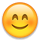## Forum Stats

• 3,727,616 Users
• 2,245,418 Discussions

Discussions

#### Howdy, Stranger!

It looks like you're new here. If you want to get involved, click one of these buttons!

# Something is wrong with my loops. Could someone help me?

Hello. This is my first time in this forum. I'm a 15 year old kid trying to learn Java from the internet. So sorry for some dumb questions I may ask(This may be one of those, too.)

I was trying to make a program that:

1. Converts Binary numbers to Decimal Numbers
2. Calculates the sum
3. Shows the outcome in both binary and in decimal

When I was almost finishing the code that does the first step, I had a problem.

The program was supposed to divide the binary numbers to columns and add the power of 2 (2^0 2^1 and so on) to the result if the column was 1 and not 0, but what happens is that the second column is acting weird,

and when it is 1 it adds 1 instead of 2 (its the second column so it was supposed to add 2^1's result)

Then I added some println in my code so i could have a better understanding of what was happening with my loops, but I can't figure out the problem. (Maybe the problem is not even in the loops but in something else.)

package binary2decimal2binary;

import java.util.Scanner;

import java.util.ArrayList;

public class Binary2Decimal2Binary {

private static long binary1;

private static long binary2;

private final static ArrayList POWERSOF2 = new ArrayList<Integer>();

private final static ArrayList<Integer> SEPARATED2 = new ArrayList<Integer>();

private static int result1 = 0;

private static int result2 = 0;

private final static ArrayList<Integer> SEPARATED1 = new ArrayList<Integer>();

static void binary2ArrayList(long binary1, long binary2){

String separating1 = String.valueOf(binary1);

for(int i = 0; i < separating1.length(); i++) {

int j =(int) Character.digit(separating1.charAt(i), 10);

String separating2 = String.valueOf(binary2);

for(int x = 0; x < separating2.length(); x++) {

int y =(int) Character.digit(separating2.charAt(x), 10);

}

System.out.println("Debugger 1 :" + SEPARATED1);

System.out.println("Debugger2 :" + SEPARATED2);

Arraylist2Decimal();

}

static void Arraylist2Decimal(){

for(int i = 0; i < SEPARATED1.size(); i++) {

int intValue = SEPARATED1.get(i);

if(intValue == 1){

result1 += (int) POWERSOF2.get(i);

}

System.out.println("Debugger 3:" + result1);

}

for(int k = 0; k < SEPARATED2.size(); k++) {

int intValue = SEPARATED2.get(k);

if(intValue == 1){

result2 += (int) POWERSOF2.get(k);

}

System.out.println("Debugger 4 :" + result2);

}};

public static void main(String[] args) {

System.out.println("Powers of 2:" + POWERSOF2);

Scanner scan = new Scanner(System.in);

System.out.println("Input first binary number.");

binary1 = scan.nextLong();

System.out.println("Input second binary number.");

binary2 = scan.nextLong();

scan.close();

binary2ArrayList(binary1,binary2);

System.out.println("First Number Converted:" + result1);

System.out.println("Second Number Converted:" + result2);

}

Inputs + Outputs:

Powers of 2:[1, 2, 4, 8, 16, 32, 64, 128, 256, 512, 1024, 2048, 4096, 8192, 16384, 32768]

Input first binary number.

(Input) 101

Input second binary number.

(Input) 10

Debugger 1 :[1, 0, 1]

Debugger2 :[1, 0]

Debugger 3:1

Debugger 3:1

Debugger 3:5

Debugger 4 :1

Debugger 4 :1

First Number Converted: 5

Second Number Converted: 1

}

Second attempt:

Powers of 2:[1, 2, 4, 8, 16, 32, 64, 128, 256, 512, 1024, 2048, 4096, 8192, 16384, 32768]

Input first binary number.

10

Input second binary number.

11

Debugger 1 :[1, 0]

Debugger2 :[1, 1]

Debugger 3:1

Debugger 3:1

Debugger 4 :1

Debugger 4First Number Converted: 1

Second Number Converted: 3

Something is wrong with the output when I input 10. I would be grateful if someone could help me.

Tagged:

•Member, Moderator Posts: 7,147 Silver Trophy

Hi,

your conversion from binary to int doesn't work. It only works for palindrome numbers. when you convert 10 you check the 1 first then the 0. The for loop needs to run backwards, or you have to do some calculations for the lookup in POWERSOF2.

Hint: look at the Long API, spaecifically Long.parseLong(...)

Regards, Phil

• Sorry for the late reply.

Thanks for the answer! But now I have another problem in my code.

I changed the

int j =(int) Character.digit(separating1.charAt(i), 10);

to

int j =(int) Character.digit(separating1.charAt(separating1.length() - i), 10);

but now my code throws a StringIndexOutOfBoundsException.

here is the error message.

Exception in thread "main" java.lang.StringIndexOutOfBoundsException: String index out of range: 3

at java.lang.String.charAt(String.java:658)

at binary2decimal2binary.Binary2Decimal2Binary.binary2ArrayList(Binary2Decimal2Binary.java:19)

at binary2decimal2binary.Binary2Decimal2Binary.main(Binary2Decimal2Binary.java:79)

Could you help me one last time?

• Hi,

given your input is 110, then separating1.length() - i is 3 at the first run of your loop. 3 is out of range as the highest index is 2 ( zero based). You need to substract a 1.

int j =(int) Character.digit(separating1.charAt(separating1.length() - i - 1), 10);

Regards, Phil

• `Could you help me one last time?`

Create a new thread for new questions.

You need to learn how to troubleshoot and debug code for yourself.

There are MANY tutorials and examples on the web on how to use a tool like NetBeans to step through code one line at a time so you can examine variables and see what the code is doing.

This discussion has been closed.